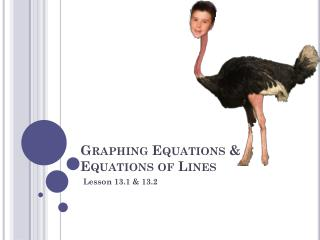Download PresentationGraphing Equations & Equations of Lines# Graphing Equations & Equations of Lines - PowerPoint PPT Presentation

Download Presentation##### Graphing Equations & Equations of Lines

Download Policy: Content on the Website is provided to you AS IS for your information and personal use and may not be sold / licensed / shared on other websites without getting consent from its author. While downloading, if for some reason you are not able to download a presentation, the publisher may have deleted the file from their server.

- - - - - - - - - - - - - - - - - - - - - - - - - - - E N D - - - - - - - - - - - - - - - - - - - - - - - - - - -
##### Presentation Transcript

1. Graphing Equations & Equations of Lines Lesson 13.1 & 13.2

2. Strategies to Graph a Line • Make a table. • Special lines • Vertical lines • Horizontal Lines • Intercepts (x, 0) & (0, y) • Slope-intercept form

3. Make a Table & Graph 2x – 4y = 10 -3 -5/2 -2 -3/2

4. Special lines:Graph y = 4 & x = -2 y = 4 is a horizontal line where all the y values are 4. x = -2 is a vertical line where all of the x values are -2.

5. InterceptsFind the x- and y- intercepts for the line 2x – 5y = 10, then graph. X –intercept: What is x when y is zero? 2x – 5(0) = 10 x = 5 …. (5, 0) Y-intercept: What is y when x is zero? 2(0) – 5y = 10 y = -2 … (0, -2)

6. Use Slope-Intercept Form to Graph the Line y = 2x - 5. • y = mx + b • y & x are any point (x, y) that line on the line. • m is the slope • b is the y-intercept (0, b) • In the equation y = 2x – 5, • m = 2 • b = (0, -5) • Graph the y-intercept • Graph the slope • Connect the dots.

7. Various Forms of Linear Equations • Slope-Intercept Form (y-form) • y = mx + b • Point-Slope Form • y – y1 = m(x – x1) • m = slope and (x1, y1) is a known point • Standard Form (General Form) • Ax + By = C

8. Things NOT to Forget about Linear Equations • Parallel Lines have the SAME slope but different y-intercepts. • Perpendicular lines have slopes that are negative reciprocals. • Vertical lines have a slope that is undefined. • Horizontal lines have a slope of zero. • Midpoint between two points is found using the formula: x1 + x2 , y1 + y2 2 2